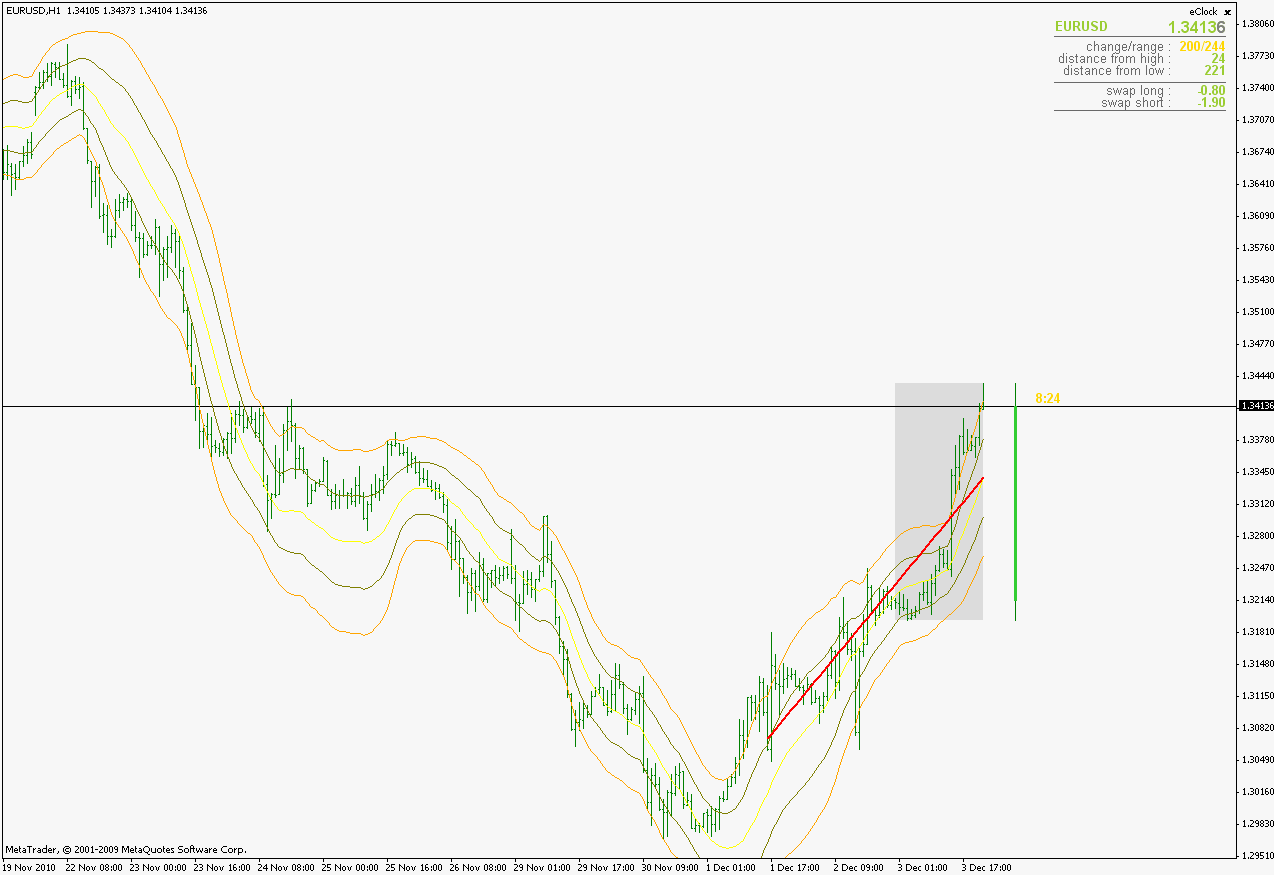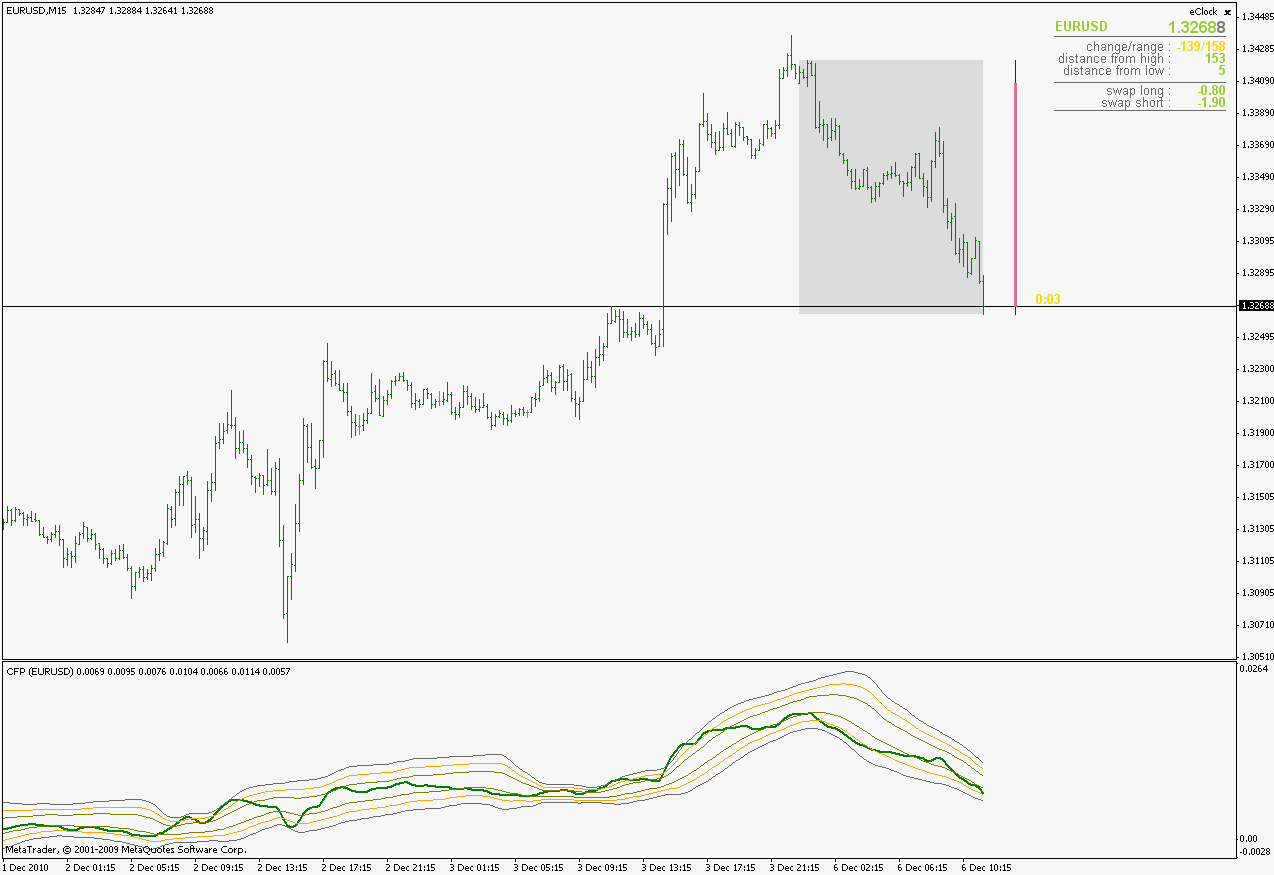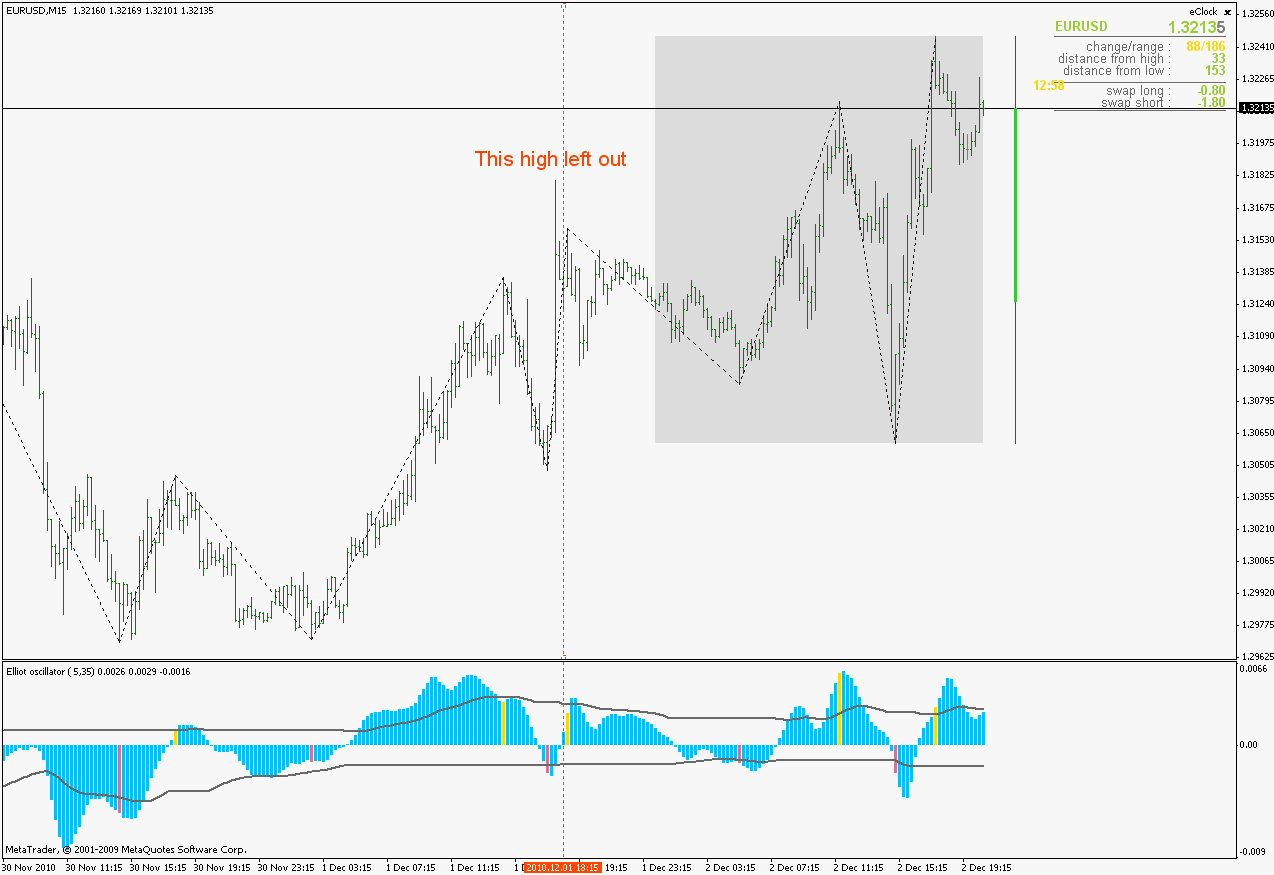• Get access

# Requests & Ideas - page 31118

Bump - Regression and deviation - line slope?

...

I assume the slope of the regression line is LinearRegSlope.

Is there an indicator buffer available to expose the slope of the regression line or are they all consumed?

The regression line slope would be nice to know as it can be used (1) to indicate direction resulting from regression as opposed to moving average, (2) compare against a preset to aid in a decision to take a trade if Price Action has made it to the n-standard deviation, (3) signal a reversal breakout if PA moves back across the line and so forth.

...

This is a bump of the previous message.

Two things:

(1) Could you take away the 3rd channel and free up a buffer to expose the slope? or add a switch that does this?

(2) While I was trying to accomplish this, I noticed...

double slope;

double value = iLRValue(slope,LR.length,i);

double std.dev = 0;

for(int k=0; k<LR.length; k++)

{

double diff = prices-(value-k*slope);

It looks like slope is declared, passed as a parameter and then used in a calculation without an assignment - is this correct?

Thanks,163385

Just the part about the slope now :

The slope parameter is passed by reference. In the declaration of the function
double iLRValue(double& LinearRegSlope, int len, int shift) [/php]
the "&" before LinearRegSlopeparameter means that it is receiving a reference to a parameter, and referenced parameters are accessible from the function itself and can be changed (assigned values that will be "seen" from the calling code). In the iLRValue function slope is calculated as one of the steps of calculating linear regression value, so this manner simply saves calculation time, since the slope parameter is assigned value in the function and after that the function returns the value of the linear regression value. Otherwise it would take 2 functions or 2 function calls with basically same calculation

So don't worry, it is not a mistake, just a little "dirty trick"If you remove the "&" from function declaration then the slope would be a constant 0 all the time and then it would be a mistake.

regards

This is a bump of the previous message.

Two things:

(1) Could you take away the 3rd channel and free up a buffer to expose the slope? or add a switch that does this?

(2) While I was trying to accomplish this, I noticed...

[php] double slope;

double value = iLRValue(slope,LR.length,i);

double std.dev = 0;

for(int k=0; k<LR.length; k++)

{

double diff = prices-(value-k*slope);

It looks like slope is declared, passed as a parameter and then used in a calculation without an assignment - is this correct?

Thanks,163385

And the second partSlope line added and removed the 2 outer regression linesregards

Files:118

...So don't worry, it is not a mistake, just a little "dirty trick"If you remove the "&" from function declaration then the slope would be a constant 0 all the time and then it would be a mistake.

regards

Got it; thanks for the coding lesson.

I deleted the 3rd channel stuff and re-purposed one buffer and tried...

mean.Buffer = value;

high.1.Buffer = mean.Buffer+(std.channel.1*std.dev);

low.1.Buffer = mean.Buffer-(std.channel.1*std.dev);

high.2.Buffer = mean.Buffer+(std.channel.2*std.dev);

low.2.Buffer = mean.Buffer-(std.channel.2*std.dev);

slope.Buffer = slope;

There were no errors, but nothing displayed either - so, it was based on this that I thought perhaps there was a 0 or null value for slope.

I look forward to seeing how it should really be done...163385

Slope line is calculated as a current linear regression value - slope*count for count = 0 to Linear regression length (it is a difference per bar (not even an angle as some think)) So slope is a small value that did not show on your chart (or better told, it did but it was somewhere around 0 and it was not visible at a normally scaled chart)

Already posted it in the previous post, but made one change in it in the mean time : in the mtf mode there was an unpleasant "down line" at the starting point of the slope line - it is corrected now

regards118

...Already posted it in the previous post, but made one change in it in the mean time : in the mtf mode there was an unpleasant "down line" at the starting point of the slope line - it is corrected now

regards

Yowsah! A double, grand slam - slope and mtf! Thanks!

'tis always nice when you know what you're doing.163385

biddick

Here you goIn this one the linear regression value itself is left out (thought that it would be "more readable" this way)regards

biddick:
Mladen, Is it possible for you to code CFP indicator with regression lines since CFP indicator has some interesting features compare to raw price https://www.mql5.com/en/forum/179807/page19 ? Many thanks.
Files:339

Continuation of the Elliot oscillator - waves indicator

______________________

What has been done :
No limit in waves (now it draws waves for the whole history) Changed a logic a bit. It affects the way how extremes are found in some cases. The basic logic is the following : when (if) the oscillator value breaks the EMA value up or down then a new wave is started if the direction of the cross is oposite to the current wave direction (crossing zero line does not start a wave nor it ends the current wave). The beginning of the wave is at the first value belonging to that wave that crosses the zero value in the direction of the wave. Here is an example : the highest bar is not marked as extreme simply because it was still not confirmed by the oscillator value that it belongs to up wave.Optimized the work (it is faster now - it calculates effectively just the last leg of the wave (it is necessary because of the changes that can happen to the last leg))
______________________ Also, as a note : only the last leg of the wave can change if new high or new low is reached. Once it is "opened" it can not (and I repeat) it can not disappear (like in some zigzags or some peak finding indicators), it can only go higher or lower if the price moves in the right direction. Its starting point is always going to stay fixed. So in combination with the oscillator itself I think it can be a useful tool

I have found similar work in mql codebase, It may give you additional ideas MACD с адаптивным определением ценовых экстремумов. - MQL4 Code Base , the problem is it is in Russian(google translation is bad) and Macd extreme indicator doesnt show any extreme levels(standart deviation or ATR ) at all.Can you have a look please? Regards.

Files:
mcd_extr..png 94 kb163385

Files:339

Thank you for debugging my headMladen.